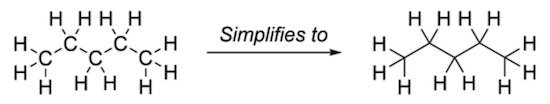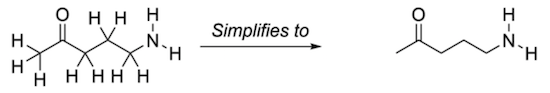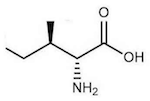Skeletal Formula Notations

This section provides a quick introduction on what is atomic bond that ties atoms together to form molecules.

There are a number of notations are used Skeletal Formula to simiplify molecule diagrams.

1. Carbon Element Symbols Removed - Since carbon elements are so common in molecules, carbon element symbols are removed from Skeletal Formula diagrams. When you see a bond end point without element symbol, you can assume that it is a carbon element.Skeletal Diagram with Carbon Symbol Removed

2. Hydrogen Elements and Bonds Removed - Since bonds between hydrogen elements and carbon Elements are so common in molecules, all hydrogen-carbon bonds are removed from Skeletal Formula diagrams. When you see a carbon element missing bonds, you can assume that it has additional bonds with hydrogen elements.Skeletal Diagram with Hydrogen Element Removed

3. Wedge and Dash Projections - If 3 dimensional information needs to be maintained in Skeletal Formula diagrams, you can use Wedge and Dash projections. A wedge projection indicates a bond that pointing forward towards the viewer. A dash projection indicates a bond that pointing away from the viewer.Skeletal Diagram with Wedge and Dash Projections

4. Condensed Branches - Some simple branches can be written as condensed Formulas like OH, NH2.Skeletal Diagram with Condensed Branches

Table of Contents